Mrs R King

•Translation

Activity

Mrs R King

•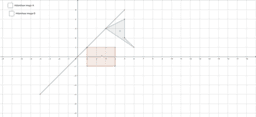Reflection on a grid

Activity

Mrs R King

•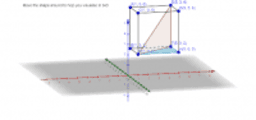3-D Pythagoras and Trigonometry exploration

Activity

Mrs R King

•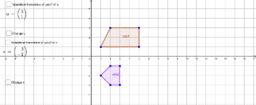Translation

Activity

Mrs R King

•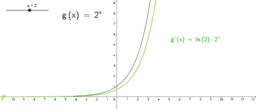Exponential demo

Activity

Mrs R King

•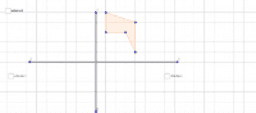Reflection in 2 lines

Activity

Mrs R King

•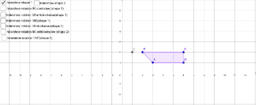Rotation on a grid

Activity

Mrs R King

•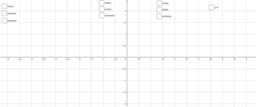Trig graphs and inverses

Activity

Mrs R King

•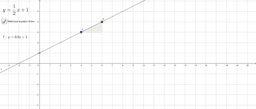Equation of line between 2 points

Activity

Mrs R King

•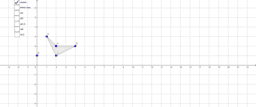Enlargement

Activity

Mrs R King

•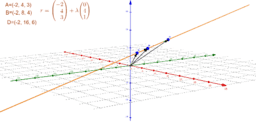Finding the angle between 2 vector lines

Activity

Mrs R King

•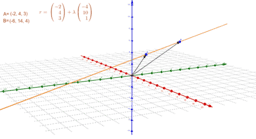Vector equation of a line in 3-D

Activity

Mrs R King

•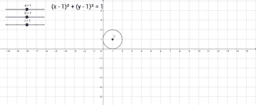Equation of a circle

Activity

Mrs R King

•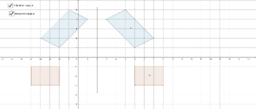Reflection on a grid

Activity

Mrs R King

•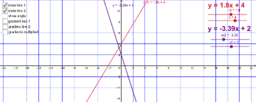Straight line graphs including perpendicular demo

Activity

Mrs R King

•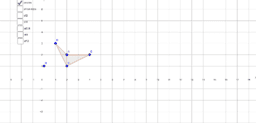Activity

Mrs R King